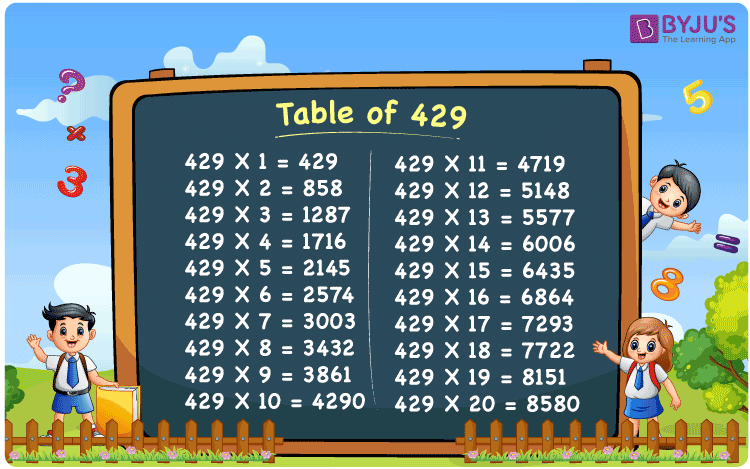Checkout JEE MAINS 2022 Question Paper Analysis : Checkout JEE MAINS 2022 Question Paper Analysis :

# Table of 429

The Table of 429 provides the results gained by repeated addition of number 429 for a certain number of times. Multiplication tables are the basics in Mathematics. Solving the problems with the help of multiplication tables boosts problem-solving and time management skills, which are essential from an exam point of view. Get the PDF of table 429 at BYJU’S, for a better academic performance.## What is the 429 Times Table?

The repeated addition of the number 429 represents the multiplication table of 429. The table of 429 is given here for ease of learning. Students can learn the table of 429 to solve the problems in a quick way.

 429×1 = 429 429 429×2 = 858 429 + 429 = 858 429×3 = 1287 429 + 429 + 429 = 1287 429×4 = 1716 429 + 429 + 429 + 429 = 1716 429×5 = 2145 429 + 429 + 429 + 429 + 429 = 2145 429×6 = 2574 429 + 429 + 429 + 429 + 429 + 429 = 2574 429×7 = 3003 429 + 429 + 429 + 429 + 429 + 429 + 429 = 3003 429×8 = 3432 429 + 429 + 429 + 429 + 429 + 429 + 429 + 429 = 3432 429×9 = 3861 429 + 429 + 429 + 429 + 429 + 429 + 429 + 429 + 429 = 3861 429×10 = 4290 429 + 429 + 429 + 429 + 429 + 429 + 429 + 429 + 429 + 429 = 4290

## Multiplication Table of 429

A multiplication table of 429 is given here for students who are in search of 429 multiples. This table will help students to solve the problems quickly.

 429 × 1 = 429 429 × 2 = 858 429 × 3 = 1287 429 × 4 = 1716 429 × 5 = 2145 429 × 6 = 2574 429 × 7 = 3003 429 × 8 = 3432 429 × 9 = 3861 429 × 10 = 4290 429 × 11 = 4719 429 × 12 = 5148 429 × 13 = 5577 429 × 14 = 6006 429 × 15 = 6435 429 × 16 = 6864 429 × 17 = 7293 429 × 18 = 7722 429 × 19 = 8151 429 × 20 = 8580

## Solved Example on the Table of 429

Example 1:

Find the value of 429 times 15 plus 50.

Solution:

429 times 15 plus 50

= 429 x 15 + 50

= 6435 + 50

= 6485

## Frequently Asked Questions on Table of 429

### What are the prime factors of 429?

3, 11, 13 are the prime factors of 429.

### Evaluate 429 times 11 minus 50 plus 70.

429 times 11 minus 50 plus 70
= 429 x 11 – 50 + 70
= 4719 – 50 + 70
= 4739

### List the first five multiples of 429.

The first five multiples of 429 are 429, 858, 1287, 1716, and 2145.